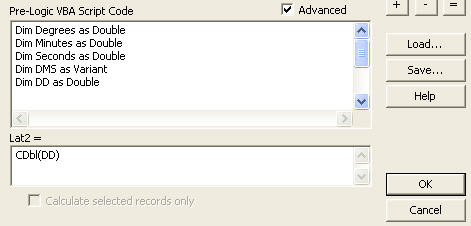English

# How To: Convert degrees minutes seconds values to decimal degree values using the Field Calculator

## Summary

```Warning:
The procedure below is for ArcGIS 9.3.1 or earlier versions. Starting at ArcGIS 10.0, expressions in the Calculator are created using only VBScript or a standard Python format.
```

Instructions provided describe how to use the Field Calculator to convert Degrees Minutes Seconds stored in a string field to Decimal Degrees stored in a number field. The values must be stored in a field in a table as Degrees Minutes Seconds with no symbols. For example:

25 35 22.3

In the example, 25 is degrees, 35 is minutes and 22.3 is seconds.

## Procedure

```Note:
Initially perform the steps below on positive numbers. After the conversion is complete, multiple any fields that need to be negative by -1.```
```Note:
The format of the records within the Degrees/Minutes/Second fields must be DD MM SS. Any blank records or records with extra spaces or characters, such as single or double quotes, may cause a user interrupted error.```
1. Add the table to ArcMap.
3. Verify 'Edit' mode is not enabled. Click the Options button and select Add Field.
4. Enter Lat2 in the Name field and select Double from the Type drop-down list. If Lat2 is already used as a field name, select a name that is not used.
5. Verify that the Scale and Precision is set to 0 and click OK.
6. Right-click on the Lat2 field and select Calculate Values.
7. Click Yes if presented with a message box.
8. Check the Advanced check box.
9. Paste the following code into the Pre-Logic VBA box:
```Code:
Dim Degrees as Double
Dim Minutes as Double
Dim Seconds as Double
Dim DMS as Variant
Dim DD as Double

DMS =  Split([Latitude])
Degrees = CDbl(DMS(0))
Minutes = CDbl(DMS(1))
Seconds = CDbl(DMS(2))
DD = (Seconds/3600) + (Minutes/60)+ Degrees```
1. Find the line that begins 'DMS. . .' The text within the brackets [ ] is the name of the field holding the latitude values. Replace the word Latitude in the code with the name of the field that stores the latitude values in the table.
2. Paste the following code into the 'Lat2 =' box at the bottom of the dialog box.
```Code:
CDbl(DD)```1. Click OK.
2. Repeat steps 3 through 12 for the longitude values.
```Note:
Null values in the DMS field will cause an error when running the VBA code in the Field Calculator.```